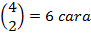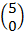-->

# Binomial Distribution Calculations In Matlab

Before practicing the calculation of the Binom distribution to MATLAB. We should discuss about the Variety of Bernoulli and Binom so that we understand the benefits of binomic calculations applied to MATLAB software.

Suppose we do an experiment whose results can be classified as "success" "failure". If we take X = 1 to declare success and X = 0 for failure, then the function of the chance mass is

A random X variable is called the Bernoulli random variable (named after the Swiss mathematician, James Bernoulli) if the mass function is given by Equation (1) for a certain value.

Now suppose we will do n free actions, each of which has the chance p to "succeed" and 1-p to "fail". If X states how many times there have been successes in these actions, then X is called the Binom Random Variable with the parameter (n, p). So, the Bernoulli random variable is nothing but a binomic random variable with parameters (1, p).

The probability mass function for a binom random variable with parameter (n, p) is

Equation (2) can be verified first by noting that the probability of a particular sequence of results of experiments containing success and failure is based on the assumption that actions are free or unbound,.

different lines n the results of experiments that produce i success and n-i failure, then prove equation (2).

As an illustration, if n = 4, i = 2, then there areways, of the four free actions produce two successes, namely

(b,b,g,g),(b,g,b,g),(b,g,g,b),(g,b,b,g),(g,b,g,b),(g,g,b,b)

in this case, for example, (b, b, g, g) means that the first two actions result in success and the last two failures. Because every result of this experiment is likely to occur as big asthen the chances of two successes from the four free actions are.

Note that according to the binomic theorem, the sum of all these opportunities is equal to one in other words,

Examples of Binom Distribution Probability Calculations in MATLAB
Five equilibrium coins were thrown. If the experimental results are assumed to be free, determine the mass function of the opportunity for the number of sides of the image that appears.

Settlement; If X states the number of sides of the image ("success") that appear, then X is a binomic random variable with parameters (n = 5, p = 1/2). Therefore, according to Equation 2.

Note: n the number 0 results 1, and 0 factorial results 1, thecalculation uses a combination calculation because the arrangement of images does not pay attention to the sequence.

Now we apply it to the calculation in MATLAB. Where we will match the results of the manual calculation above with calculations using MATLAB, whether the results are the same and make a plot (bar diagram) of the probability period function of the binomial distribution with n = 5 and p = 0.5, writing the syntax in the Command Window is,

% Specifies the value of X
x = 0: 5;
% Describes the plot of the probable function period of the binomial distribution
% First for n = 5, p = 0.5
pdf = binopdf (x, 5,0.5)

pdf =

0.0313    0.1562    0.3125    0.3125    0.1562    0.0313

subplot (1,2,1), bar (x, pdf)
title ('n = 5, p = 0.5')
xlabel ('X'), ylabel ('f (X)')
axis square